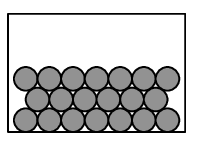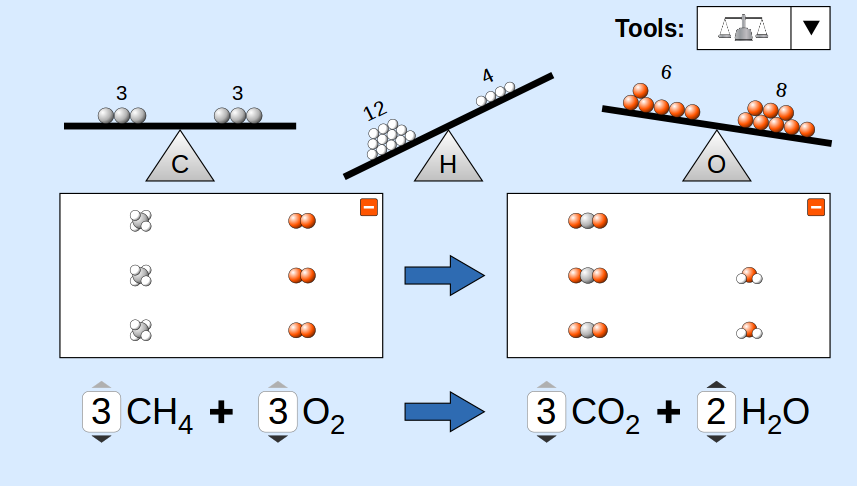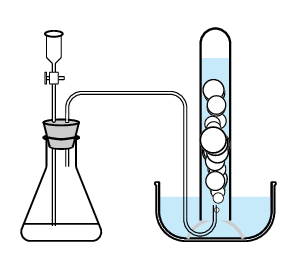Home Practice
For learners and parents For teachers and schools
Textbooks
Full catalogue
Pricing SupportLog in

We think you are located in United States. Is this correct?

# 14.2 Balancing chemical equations

## 14.2 Balancing chemical equations (ESAEB)

### The law of conservation of mass (ESAEC)

In order to balance a chemical equation, it is important to understand the law of conservation of mass.

The law of conservation of mass

The mass of a closed system of substances will remain constant, regardless of the processes acting inside the system. Matter can change form, but cannot be created or destroyed.

For any chemical equation (in a closed system) the mass of the reactants must be equal to the mass of the products. In order to make sure that this is the case, the number of atoms of each element in the reactants must be equal to the number of atoms of those same elements in the products. An example is shown below:

Iron is a metal. When we represent it in a balanced chemical equation, we write only $$\text{Fe}$$. Sulfur occurs as $$\text{S}_{8}$$ but we write only the empirical formula: $$\text{S}$$. We do this for all network structures. Writing formulae like this represents one unit of the compound or network structure.$$\text{Fe} + \text{S} \rightarrow \text{FeS}$$ Mass of one atom of $$\text{Fe}$$ is $$\text{55,8}$$ Mass of one atom of $$\text{S}$$ is $$\text{32,1}$$ Mass of one atom of $$\text{FeS}$$ is $$\text{87,9}$$ Mass of reactants is $$\text{87,9}$$ Mass of products is $$\text{87,9}$$

To calculate the mass of the molecules we use the relative atomic masses for iron and sulfur, as seen in the table above. You will notice that the mass of the reactants equals the mass of the product. A chemical equation that is balanced will always reflect the law of conservation of mass and the law of conservation of atoms.

temp text

## Balancing chemical equations

### 1.

You will need: coloured balls (or marbles), prestik, a sheet of paper and coloured pens.

We will try to balance the following equation:

$\text{Al} + \text{O}_{2} \rightarrow \text{Al}_{2}\text{O}_{3}$

Take one ball of one colour. This represents a molecule of $$\text{Al}$$. Take two balls of another colour and stick them together. This represents a molecule of $$\text{O}_{2}$$. Place these molecules on your left. Now take two balls of the first colour and three balls of the second colour to form $$\text{Al}_{2}\text{O}_{3}$$. Place this compound on your right. On a piece of paper draw coloured circles to represent the balls. Draw a line down the centre of the paper to represent the molecules on the left and on the right.

Count the number of balls on the left and the number on the right. Do you have the same number of each colour on both sides? If not, the equation is not balanced. How many balls of each colour will you have to add to each side to make the number of balls the same? How would you add these balls?

You should find that you need four balls of the first colour for $$\text{Al}$$ and three pairs of balls of the second colour (i.e. six balls in total) for $$)_{2}$$ on the left side. On the right side you should find that you need $$\text{2}$$ clusters of balls for $$\text{Al}_{2}\text{O}_{3}$$. We say that the balanced equation is:

$4\text{Al} + 3\text{O}_{2} \rightarrow 2\text{Al}_{2}\text{O}_{3}$

### 2.

Use jelly tots and toothpicks to build the following chemical equation. Make sure that your atoms are balanced. Use the same colour jelly tots for the same atoms.

$\text{C} + \text{H}_{2}\text{O} \rightarrow \text{CO}_{2} + \text{CO} + \text{H}_{2}$

Add compounds until the atoms are balanced. Write the equation down and use a coefficient to indicate how many compounds you used. For example if you had to use three water molecules then write $$3\text{H}_{2}\text{O}$$

### 3

Use ball and stick drawings to balance the atoms in the following reaction:

$\text{NH}_{3} + \text{O}_{2} \rightarrow \text{NO} + \text{H}_{2}\text{O}$

Use your drawings to write a balanced chemical equation for the reaction.

### 4

Lead $$(\text{Pb})$$, lead (IV) oxide $$(\text{PbO}_{2})$$ and sulfuric acid $$(\text{H}_{2}\text{SO}_{4})$$ are used in car batteries. The following reaction takes place: $$\text{Pb} + \text{PbO}_{2} + \text{H}_{2}\text{SO}_{4} \rightarrow \text{PbSO}_{4} + \text{H}_{2}\text{O}$$

Cut out circles from four different colours of paper to represent each of the atoms. Build a few of the compounds ($$\text{Pb}$$, $$\text{PbO}_{2}$$, $$\text{H}_{2}\text{SO}_{4}$$). These are the reactants. Do not build the products. Rearrange the atoms so that the products are formed. Add more reactants if needed to balance the atoms (e.g. you will need two $$\text{H}_{2}\text{SO}_{4}$$ molecules). Use what you have learnt to write a balanced equation for the reaction.### Steps to balance a chemical equation through inspection (ESAED)

When balancing a chemical equation, there are a number of steps that need to be followed.

1. Identify the reactants and the products in the reaction and write their chemical formulae.

2. Write the equation by putting the reactants on the left of the arrow and the products on the right.

3. Count the number of atoms of each element in the reactants and the number of atoms of each element in the products.

4. If the equation is not balanced, change the coefficients of the molecules until the number of atoms of each element on either side of the equation balance.

5. Check that the atoms are in fact balanced.

6. (we will look at this a little later): Add any extra details to the equation e.g. phase symbols.

temp text

## Worked example 1: Balancing chemical equations 1

Balance the following equation:

$\text{Mg} + \text{HCl} \rightarrow \text{MgCl}_{2} + \text{H}_{2}$

### Identify the reactants and products

This has been done in the question.

### Write the equation for the reaction

This has been done in the question.

### Count the number of atoms of each element in the reactants and products

Reactants: $$\text{Mg} = 1 \text{ atom}$$, $$\text{H} = 1 \text{ atom}$$, $$\text{Cl} = 1 \text{ atom}$$

Products: $$\text{Mg} = 1 \text{ atom}$$, $$\text{H} = 2 \text{ atoms}$$, $$\text{Cl} = 2 \text{ atoms}$$

### Balance the equation

The equation is not balanced since there are two chlorine atoms in the product and only one in the reactants. If we add a coefficient of two to the $$\text{HCl}$$ to increase the number of $$\text{H}$$ and $$\text{Cl}$$ atoms in the reactants, the equation will look like this:

$\text{Mg} + 2\text{HCl} \rightarrow \text{MgCl}_{2} + \text{H}_{2}$

### Check that the atoms are balanced

If we count the atoms on each side of the equation, we find the following:

Reactants: $$\text{Mg} = 1 \text{ atom}$$, $$\text{H} = 2 \text{ atoms}$$, $$\text{Cl} = 2 \text{ atoms}$$

Products: $$\text{Mg} = 1 \text{ atom}$$, $$\text{H} = 2 \text{ atoms}$$, $$\text{Cl} = 2 \text{ atoms}$$

The equation is balanced. The final equation is:

$\text{Mg} + 2\text{HCl} \rightarrow \text{MgCl}_{2} + \text{H}_{2}$

## Worked example 2: Balancing chemical equations 2

Balance the following equation:

$\text{CH}_{4} + \text{O}_{2} \rightarrow \text{CO}_{2} + \text{H}_{2}\text{O}$

### Count the number of atoms of each element in the reactants and products

Reactants: $$\text{C} = 1$$, $$\text{H} = 4$$, $$\text{O} = 2$$

Products: $$\text{C} = 1$$, $$\text{H} = 2$$, $$\text{O} = 3$$

### Balance the equation

If we add a coefficient of $$\text{2}$$ to $$\text{H}_{2}\text{O}$$, then the number of hydrogen atoms in the products will be $$\text{4}$$, which is the same as for the reactants. The equation will be:

$\text{CH}_{4} + \text{O}_{2} \rightarrow \text{CO}_{2} + 2\text{H}_{2}\text{O}$

### Check that the atoms balance

Reactants: $$\text{C} = 1$$, $$\text{H} = 4$$, $$\text{O} = 2$$

Products: $$\text{C} = 1$$, $$\text{H} = 4$$, $$\text{O} = 4$$

You will see that, although the number of hydrogen atoms now balances, there are more oxygen atoms in the products. You now need to repeat the previous step. If we put a coefficient of $$\text{2}$$ in front of $$\text{O}_{2}$$, then we will increase the number of oxygen atoms in the reactants by $$\text{2}$$. The new equation is:

$\text{CH}_{4} + 2\text{O}_{2} \rightarrow \text{CO}_{2} + 2\text{H}_{2}\text{O}$

When we check the number of atoms again, we find that the number of atoms of each element in the reactants is the same as the number in the products. The equation is now balanced.

## Worked example 3: Balancing chemical equations 3

In our bodies, sugar $$(\text{C}_{6}\text{H}_{12}\text{O}_{6})$$ reacts with the oxygen we breathe in to produce carbon dioxide, water and energy. Write the balanced equation for this reaction.

### Identify the reactants and products in the reaction.

Reactants: sugar $$(\text{C}_{6}\text{H}_{12}\text{O}_{6})$$ and oxygen $$(\text{O}_{2})$$

Products: carbon dioxide $$(\text{CO}_{2})$$ and water $$(\text{H}_{2}\text{O})$$

### Write the equation

$\text{C}_{6}\text{H}_{12}\text{O}_{6} + \text{O}_{2} \rightarrow \text{CO}_{2} + \text{H}_{2}\text{O}$

### Count the number of atoms of each element in the reactants and in the products

Reactants: $$\text{C} = 6$$, $$\text{H} = 12$$, $$\text{O} = 8$$

Products: $$\text{C} = 1$$, $$\text{H} = 2$$, $$\text{O} = 3$$

### Balance the equation

It is easier to start with carbon as it only appears once on each side. If we add a $$\text{6}$$ in front of $$\text{CO}_{2}$$, the equation looks like this:

$\text{C}_{6}\text{H}_{12}\text{O}_{6} + \text{O}_{2} \rightarrow 6\text{CO}_{2} + \text{H}_{2}\text{O}$

Reactants: $$\text{C} = 6$$, $$\text{H} = 12$$, $$\text{O} = 8$$

Products: $$\text{C} = 6$$, $$\text{H} = 2$$, $$\text{O} = 13$$

### Change the coefficients again to try to balance the equation.

Let us try to get the number of hydrogens the same this time.

$\text{C}_{6}\text{H}_{12}\text{O}_{6} + \text{O}_{2} \rightarrow 6\text{CO}_{2} + 6\text{H}_{2}\text{O}$

Reactants: $$\text{C} = 6$$, $$\text{H} = 12$$, $$\text{O} = 8$$

Products: $$\text{C} = 6$$, $$\text{H} = 12$$, $$\text{O} = 18$$

### Now we just need to balance the oxygen atoms.

$\text{C}_{6}\text{H}_{12}\text{O}_{6} + 6\text{O}_{2} \rightarrow 6\text{CO}_{2} + 6\text{H}_{2}\text{O}$

Reactants: $$\text{C} = 6$$, $$\text{H} = 12$$, $$\text{O} = 18$$

Products: $$\text{C} = 6$$, $$\text{H} = 12$$, $$\text{O} = 18$$

## Balancing simple chemical equations

Textbook Exercise 14.2

Balance the following equations:

$\text{Mg} + \text{O}_{2} \rightarrow \text{MgO}$
Solution not yet available
$\text{Ca} + \text{H}_{2}\text{O} \rightarrow \text{Ca(OH)}_{2} + \text{H}_{2}$
Solution not yet available
$\text{CuCO}_{3} + \text{H}_{2}\text{SO}_{4} \rightarrow \text{CuSO}_{4} + \text{H}_{2}\text{O} + \text{CO}_{2}$
Solution not yet available
$\text{CaCl}_{2} + \text{Na}_{2}\text{CO}_{3} \rightarrow \text{CaCO}_{3} + \text{NaCl}$
Solution not yet available
$\text{C}_{12}\text{H}_{22}\text{O}_{11} + \text{O}_{2} \rightarrow \text{CO}_{2} + \text{H}_{2}\text{O}$
Solution not yet available

Barium chloride reacts with sulfuric acid to produce barium sulfate and hydrochloric acid.

Solution not yet available

Ethane $$(\text{C}_{2}\text{H}_{6})$$ reacts with oxygen to form carbon dioxide and steam.

Solution not yet available

Ammonium carbonate is often used as a smelling salt. Balance the following reaction for the decomposition of ammonium carbonate: $$\text{(NH}_{4}\text{)}_{2}\text{CO}_{3}\text{(s)} \rightarrow \text{NH}_{3}\text{(aq)} + \text{CO}_{2}\text{(g)} + \text{H}_{2}\text{O (l)}$$

Solution not yet available

Hydrogen fuel cells are extremely important in the development of alternative energy sources. Many of these cells work by reacting hydrogen and oxygen gases together to form water, a reaction which also produces electricity. Balance the following equation: $$\text{H}_{2}\text{(g)} + \text{O}_{2}\text{(g)} \rightarrow \text{H}_{2}\text{O (l)}$$

Solution not yet available

The synthesis of ammonia $$(\text{NH}_{3})$$, made famous by the German chemist Fritz Haber in the early 20th century, is one of the most important reactions in the chemical industry. Balance the following equation used to produce ammonia: $\text{N}_{2}\text{(g)} + \text{H}_{2}\text{(g)} \rightarrow \text{NH}_{3}\text{(g)}$

Solution not yet available

### State symbols and other information (ESAEE)

The state (phase) of compounds can be expressed in the chemical equation. This is done by placing the correct label on the right hand side of the formula. The following four labels can be used:

1. (g) for gaseous compounds

2. (l) for liquids

3. (s) for solid compounds

4. (aq) for an aqueous (water) solution

To show that heat is needed for a reaction, a Greek delta (Δ) is placed above the arrow. For example: $$\text{NH}_{4}\text{Cl} \stackrel{\Delta}{\rightarrow} \text{NH}_{3} + \text{HCl}$$

temp text

## Worked example 4: Balancing chemical equations 4

Solid zinc metal reacts with aqueous hydrochloric acid to form an aqueous solution of zinc chloride $$(\text{ZnCl}_{2})$$ and hydrogen gas. Write a balanced equation for this reaction.

### Identify the reactants and products

The reactants are zinc $$(\text{Zn})$$ and hydrochloric acid $$(\text{HCl})$$. The products are zinc chloride $$(\text{ZnCl}_{2})$$ and hydrogen $$(\text{H}_{2})$$.

### Write the equation

$\text{Zn} + \text{HCl} \rightarrow \text{ZnCl}_{2} + \text{H}_{2}$

### Balance the equation

You will notice that the zinc atoms balance but the chlorine and hydrogen atoms do not. Since there are two chlorine atoms on the right and only one on the left, we will give $$\text{HCl}$$ a coefficient of $$\text{2}$$ so that there will be two chlorine atoms on each side of the equation.

$\text{Zn} + 2\text{HCl} \rightarrow \text{ZnCl}_{2} + \text{H}_{2}$

### Check that all the atoms balance

When you look at the equation again, you will see that all the atoms are now balanced.

### Ensure all details (e.g. state symbols) are added

In the initial description, you were told that zinc was a metal, hydrochloric acid and zinc chloride were in aqueous solutions and hydrogen was a gas.

$\text{Zn (s)} + \text{HCl (aq)} \rightarrow \text{ZnCl}_{2}\text{(aq)} + \text{H}_{2}\text{(g)}$

## State symbols

Textbook Exercise 14.3

Write balanced equations for each of the following reactions, include state symbols:

Lead (II) nitrate solution reacts with a potassium iodide solution to form a precipitate (solid) of lead iodide while potassium nitrate remains in solution.

Solution not yet available

When heated, aluminium metal reacts with solid copper oxide to produce copper metal and aluminium oxide $$(\text{Al}_{2}\text{O}_{3})$$.

Solution not yet available

When calcium chloride solution is mixed with silver nitrate solution, a white precipitate (solid) of silver chloride appears. Calcium nitrate $$(\text{Ca}(\text{NO}_{3})_{2})$$ is also produced in the solution.

Solution not yet available

Solid ammonium carbonate decomposes to form three gaseous products.

Solution not yet available

## The relationship between product and reactant

### Aim

To investigate the relationship between the amount of product and the amount of reactant.

### Apparatus

• measuring cylinder

• water bowl

• delivery tube

• funnel with stopcock

• stopper

• sodium hydrogen carbonate $$(\text{Na}(\text{HCO}_{3})$$ powder

• dilute sulfuric acid $$(\text{H}_{2}\text{SO}_{4})$$### Method

1. Weigh $$\text{20}$$ $$\text{g}$$ of $$\text{NaHCO}_{3}$$ and place it into a flask.

2. Set up the above apparatus.

3. Measure out $$\text{5}$$ $$\text{mL}$$ of $$\text{H}_{2}\text{SO}_{4}$$ and carefully pour this into the funnel (make sure that the stopcock is closed).

4. Slowly add the $$\text{H}_{2}\text{SO}_{4}$$ to the $$\text{NaHCO}_{3}$$ by opening the stopcock.

5. Observe what happens.

6. Record the volume of gas collected in the measuring cylinder.

7. Repeat the above steps but this time use $$\text{10}$$ $$\text{mL}$$ of $$\text{H}_{2}\text{SO}_{4}$$.

8. Write a balanced equation for this reaction. (Hint: carbon dioxide gas is formed, as well as water and sodium sulfate.)

### Results and discussion

You should observe that more gas is formed when using more $$\text{H}_{2}\text{SO}_{4}$$.